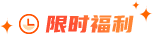### 大数据之三数之和题目描述

给定一个包含 n 个整数的数组 nums，判断 nums 中是否存在三个元素 a，b，c ，使得 a + b + c = 0 ?找出所有满足条件且不重复的三元组。

题目解析

题目需要我们找出三个数且和为 0 ，那么除了三个数全是 0 的情况之外，肯定会有负数和正数，所以一开始可以先选择一个数，然后再去找另外两个数，这样只要找到两个数且和为第一个选择的数的相反数就行了。也就是说需要枚举 a 和 b ，将 c 的存入 map 即可。

需要注意的是返回的结果中，不能有有重复的结果。这样的代码时间复杂度是 O(n^2)。在这里可以先将原数组进行排序，然后再遍历排序后的数组，这样就可以使用双指针以线性时间复杂度来遍历所有满足题意的两个数组合。

代码实现

class Solution {

public:

vector> threeSum(vector& nums) {

vector> res;

sort(nums.begin(), nums.end());

if (nums.empty() || nums.back() < 0 || nums.front() > 0) return {};

for (int k = 0; k < nums.size(); ++k) {

if (nums[k] > 0) break;

if (k > 0 && nums[k] == nums[k - 1]) continue;

int target = 0 - nums[k];

int i = k + 1. j = nums.size() - 1;

while (i < j) {

if (nums[i] + nums[j] == target) {

res.push_back({nums[k], nums[i], nums[j]});

while (i < j && nums[i] == nums[i + 1]) ++i;

while (i < j && nums[j] == nums[j - 1]) --j;

++i; --j;

} else if (nums[i] + nums[j] < target) ++i;

else --j;

}

}

return res;

}

};### 大数据师资团队更多>>

• 北京校区
• 大连校区
• 广州校区
• 成都校区
• 杭州校区
• 长沙校区
• 合肥校区
• 南京校区
• 上海校区
• 深圳校区
• 武汉校区
• 郑州校区
• 西安校区
• 青岛校区
• 重庆校区
• 太原校区
• 沈阳校区
• 南昌校区
• 哈尔滨校区